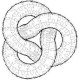The Spectre of Math

September 11, 2009

R is uncountable

Filed under: Mathematics,Teaching — jlebl @ 4:34 pm

Today I did the “$\mathbb{R}$ is uncountable” proof in class. I got a few “understanding nods”, and only one person seemed to be falling asleep (visibly). One person stormed out the moment I finished the proof, but I think it was because I went about 30 seconds over, rather than being angry at Cantor. All in all, I think that was success. Last time I did this a few years ago to a similar audience I got many angry stares. I think they were also not small Kroneckers, but more angry as in “I have to learn this nonsense?” I always find that this theorem has a quality about it that makes people either like it a lot or hate it a lot. I think much more so than anything else they will see in this class. I already had a question in office hours whose answer depended on the axiom of choice. I guess that is more subtle than uncountability of$\mathbb{R}$, but also I don’t talk about AC in class.

I did something like Cantor’s original proof of 1874. I looked at Wikipedia (which has a simplified version of Cantor’s proof) and I looked at baby Rudin, which has essentially the same proof in completely different language and in greater generality. Though I did it without using contradiction, since proofs by contradiction are evil.

Proof: Given a countable set$\{ x_1, x_2, \ldots \} \subset \mathbb{R}$, you construct a sequence of closed intervals$[a_j,b_j]$ such that$[a_{j+1},b_{j+1}] \subset (a_j,b_j)$, and such that$x_j \notin (a_{j+1},b_{j+1})$. The intersection of all the intervals is nonempty (compactness) and can’t contain any element of the countable set. QED

That’s more analysis-like than the diagonal proof, which is more computer-science-like.

1.Wow, I definitely wouldn’t be angry at being taught that…

I read Amir Aczel’s “pop-math” book on Cantor (“The mystery of the Aleph”), and despite some mumbo-jumbo in the book (Kabbala? Wtf?), I found it fascinating. I hope to someday understand that stuff properly (I mean, above the “pop-math” level..).

Comment by Mauro — September 11, 2009 @ 5:40 pm

2.… since proofs by contradiction are evil.
This leaves me puzzled since I love proofs by contradiction.
I guess it is because my wife is right and I am evil myself 🙂

And, btw, should not your proof start by saying
“Let us assume that R is countable and write it as {x1,x2,…}”
If not, I perceive a missing logical link.
If yes, it is a proof by contradiction.
Maybe you are evil, too, but you are hiding the fact artfully.

All the best, Stan

PS: nice blog; ahoj

Comment by stan — September 30, 2009 @ 9:50 am

•that’s why you’re evil 🙂 The way I said it is without contradiction. I proved that, “given a countable subset X of R, then R-X is nonempty.” Therefore R is uncountable. The reason why such a proof is less evil is that the set X is something that is just fine and exists. If you start with the contradiction statement R=X, then you are arguing about something that doesn’t exist. It’s a subtle difference.

I do contradiction proofs as well, but I find it often clearer to avoid them if possible. I like to work with things that are consistent and exist. Working with nonexistent objects makes it easier to do something wrong.

But, yes, I am evil.

Comment by jlebl — September 30, 2009 @ 5:04 pm

3.I’m not going to call this a proof, but this is quite convincing that R is not countable:

Suppose that R is countable, i.e. that R = { x(k) | k = 0, 1, 2, … }. Now cover x(k) with an interval of length 2^(-k), e.g. [x(k)-2^(-k-1), x(k)+2^(-k-1)]. Then the union of these intervals should of course cover all of R. But the total length of the intervals is just 2^(-0) + 2^(-1) + 2^(-2) + … = 2, and thus cannot cover all of R, the length of which is infinite. Hence, the assumption that R is countable is false.

Comment by Per Persson — August 6, 2010 @ 7:17 pm

•That’s actually close to a proof I guess. What’s left is to prove that a countable collection of intervals that covers R cannot have finite measure (length). That actually doesn’t seem too difficult.

Comment by jlebl — August 7, 2010 @ 2:34 am

4.Yes, we need continuity from below: If E(k) is an increasing (i.e. E(k)⊆E(k+1)) sequence of measurable sets, then m(∪E(k)) = lim m(E(k)). Here E(k) will be the union of the k first intervals.

Another remark is that we can get the measure of the union of the intervals as small as we want by taking the lengths be epsilon/2^(k+1) with epsilon small.

Comment by Per Persson — August 7, 2010 @ 12:14 pm

•even weaker thing is needed, all you need is an inequality for the measures. So subaditivity is all you need. The standard outer measure construction will suffice (that is, you need to prove that the standard outer measure defined starting with say half-open intervals is subadditive). No need to worry about measurable sets.

Comment by jlebl — August 7, 2010 @ 2:30 pm

Blog at WordPress.com.